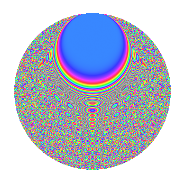# Properties

 Label 1890.2.dbLevel 1890 Weight 2 Character orbit db Rep. character $$\chi_{1890}(209,\cdot)$$ Character field $$\Q(\zeta_{18})$$ Dimension 864 Sturm bound 864

# Related objects

## Defining parameters

 Level: $$N$$ = $$1890 = 2 \cdot 3^{3} \cdot 5 \cdot 7$$ Weight: $$k$$ = $$2$$ Character orbit: $$[\chi]$$ = 1890.db (of order $$18$$ and degree $$6$$) Character conductor: $$\operatorname{cond}(\chi)$$ = $$945$$ Character field: $$\Q(\zeta_{18})$$ Sturm bound: $$864$$

## Dimensions

The following table gives the dimensions of various subspaces of $$M_{2}(1890, [\chi])$$.

Total New Old
Modular forms 2640 864 1776
Cusp forms 2544 864 1680
Eisenstein series 96 0 96

## Trace form

 $$864q + 12q^{9} + O(q^{10})$$ $$864q + 12q^{9} + 12q^{11} - 6q^{14} - 12q^{15} - 72q^{21} - 12q^{29} - 72q^{30} + 54q^{35} - 12q^{36} + 132q^{39} - 18q^{49} - 36q^{50} + 6q^{56} + 36q^{60} - 432q^{64} + 72q^{65} + 72q^{79} - 36q^{81} - 12q^{84} + 120q^{95} - 72q^{99} + O(q^{100})$$

## Decomposition of $$S_{2}^{\mathrm{new}}(1890, [\chi])$$ into newform subspaces

The newforms in this space have not yet been added to the LMFDB.

## Decomposition of $$S_{2}^{\mathrm{old}}(1890, [\chi])$$ into lower level spaces

$$S_{2}^{\mathrm{old}}(1890, [\chi]) \cong$$ $$S_{2}^{\mathrm{new}}(945, [\chi])$$$$^{\oplus 2}$$

## Hecke Characteristic Polynomials

There are no characteristic polynomials of Hecke operators in the database